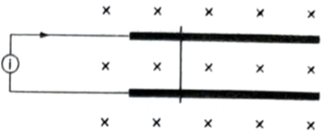Figure shows two long metal rails placed horizontally and parallel to each other at a separation l. A uniform magnetic field B exists in the vertically downward direction. A wire of mass m can slide on the rails. The rails are connected to a constant current source which drives a current i in the circuit. The friction coefficient between the rails  and the wire is µ  . What should be the minimum value of µ  which can prevent the wire from sliding on the rails?

# Figure shows two long metal rails placed horizontally and parallel to each other at a separation $l$. A uniform magnetic field B exists in the vertically downward direction. A wire of mass m can slide on the rails. The rails are connected to a constant current source which drives a current i in the circuit. The friction coefficient between the rails  and the wire is µ  . What should be the minimum value of µ  which can prevent the wire from sliding on the rails?1. A

$\frac{\mathrm{ilB}}{\mathrm{mg}}$

2. B

$\frac{2\mathrm{ilB}}{\mathrm{mg}}$

3. C

$\frac{3\mathrm{ilB}}{\mathrm{mg}}$

4. D

$\frac{4\mathrm{ilB}}{\mathrm{mg}}$

Register to Get Free Mock Test and Study Material

+91

Verify OTP Code (required)

### Solution:

The force on the wire due to the magnetic field is $\stackrel{\to }{\mathrm{F}}=\mathrm{i}\stackrel{\to }{\mathrm{l}}×\stackrel{\to }{\mathrm{B}}$
Or, $\mathrm{F}=\mathrm{ilB}$
It acts towards right in the given figure. If the wire does not slide on the rails, the force of friction by the rails should be equal to F. If ${\mathrm{\mu }}_{0}$ be the minimum coefficient of friction which can prevent sliding, this force is also equal to ${\mathrm{\mu }}_{0}$  mg. Thus,Register to Get Free Mock Test and Study Material

+91

Verify OTP Code (required)